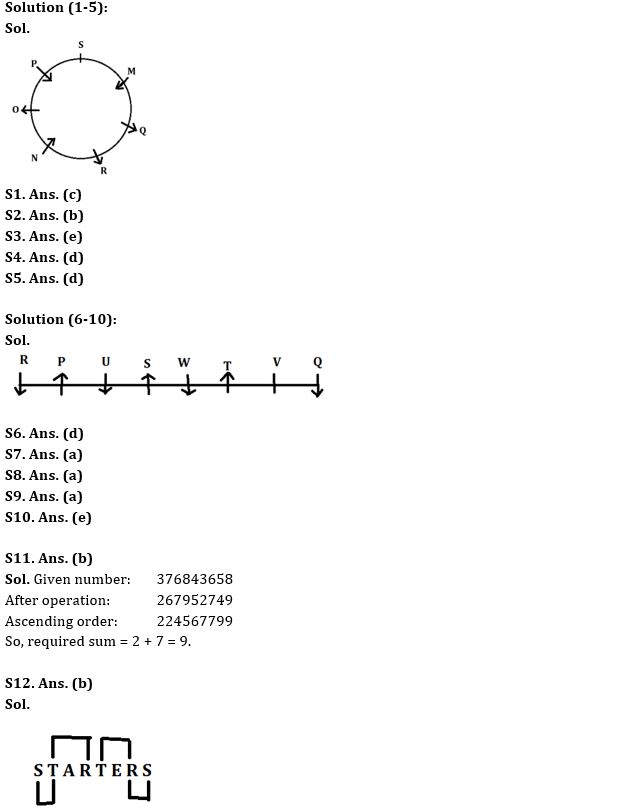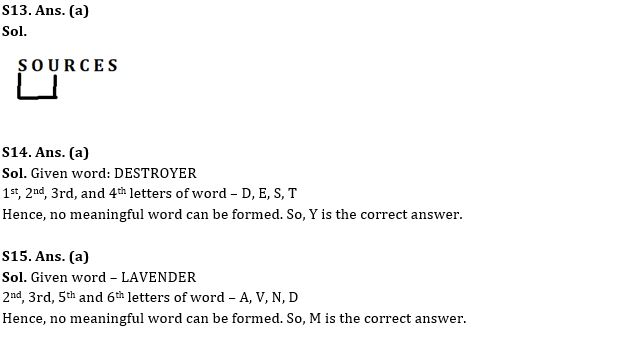Latest Banking jobs   »

# Reasoning Ability Quiz For IBPS RRB PO Clerk Prelims 2022- 9th August

Directions (1-5): Answer the questions based on the information given below.
Seven persons M, N, O, P, Q, R and S sit around the circular table facing either towards or away from the center. P sits second to the right of M. M sits second to the left of R. O and N are immediate left of each other. Q faces away from the center but does not sit adjacent to P. Persons adjacent to S face in same direction. P does not face away from the center. S does not sit second to the right of N.

Q1. Who among the following sits immediate right of R?
(a) S
(b) P
(c) N
(d) Q
(e) O

Q2. Who sits third to the right of P?
(a) S
(b) R
(c) N
(d) Q
(e) M

Q3. Who sits immediate right of S?
(a) M
(b) R
(c) N
(d) Q
(e) Can’t be determined

Q4. How many persons sit between O and Q when counted from left of O?
(a) One
(b) Four
(c) Five
(d) Two
(e) None of these

Q5. Who among the following faces same direction as Q?
(a) M
(b) P
(c) N
(d) O
(e) None of these

Directions (6-10): Answer the questions based on the information given below.
Eight persons (P, Q, R, S, T, U, V and W) are sitting in a row such that some of them are facing north and some of them are facing south. Not more than two persons sitting adjacent to each other, face same direction.
R sits to the right of U who is sitting 3rd to the left of T. R is sitting at one of the extreme ends. W is facing south and is sitting immediate left of T. T is facing the same direction as P who is sitting 3rd to the right of W. S is sitting 4th to the right of Q. S is sitting adjacent to W. Persons sitting at the extreme ends are facing same direction. U and W face the same direction. T and Q face different direction.

Q6. Who is sitting 4th to left of U?
(a) P
(b) S
(c) Q
(d) V
(e) None of these

Q7. How many persons are sitting between P and V?
(a) Four
(b) Three
(c) Two
(d) One
(e) None of these

Q8. If W is sitting 2nd to the left of V, then how many persons are facing north direction?
(a) Four
(b) Three
(c) Two
(d) Six
(e) None of these

Q9. Who among the following person is sitting immediate right of P?
(a) U
(b) R
(c) S
(d) Q
(e) None of these

Q10. Four from the following are similar in a certain way and forms a group. Find out the one, which does not belong to that group?
(a) R
(b) U
(c) Q
(d) W
(e) T

Q11. If in a given number ‘376843658‘, 1 is added to all even digits and 1 is subtracted from all odd digits and then arrange them in ascending order from left to right, then what will be the sum of third from right end and second from left end?
(a) 10
(b) 9
(c) 11
(d) 8
(e) None of the above

Q12. How many pairs of letters are there in the word ‘STARTERS’ which has as many letters between them in the word in English alphabetical series from the both forward and backward direction?
(a) Three
(b) Four
(c) Five
(d) Six
(e) None of the above

Q13. How many pairs of letters are there in the word ‘SOURCES’ which has as many letters between them in the word in English alphabetical series from the both forward and backward direction?
(a) One
(b) Four
(c) Two
(d) Three
(e) None of the above

Q14. If it is possible to make a meaningful word from 1st, 2nd, 3rd, and 4th letters of word “DESTROYER“, then which will be the 2nd letter of the meaningful word? If no such word be formed, mark the answer as ‘Y’. If more than one word can be formed mark the answer as ‘X’.
(a) Y
(b) E
(c) D
(d) S
(e) X

Q15. If it is possible to make a meaningful word from 2nd, 3rd, 5th and 6th letters of word “LAVENDER“, then which will be the 1st letter of the meaningful word? If no such word be formed, mark the answer as ‘M’. If more than one word can be formed mark the answer as ‘K’.
(a) M
(b) N
(c) D
(d) V
(e) K

Solutions#### Congratulations!Download Hindu Review of October 2021: Free PDF